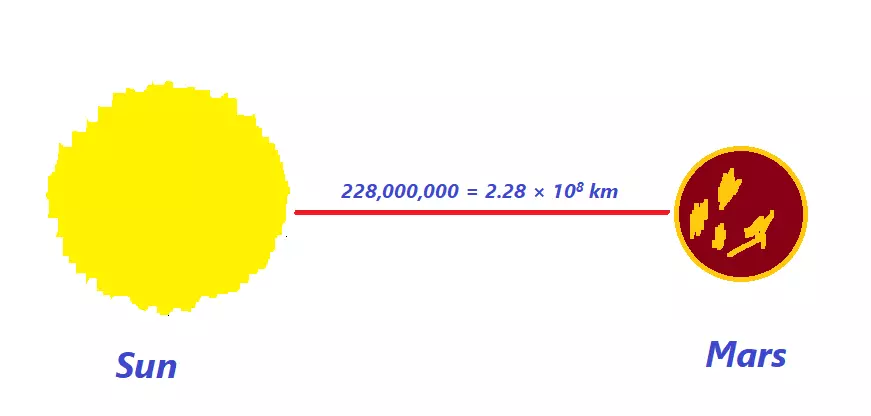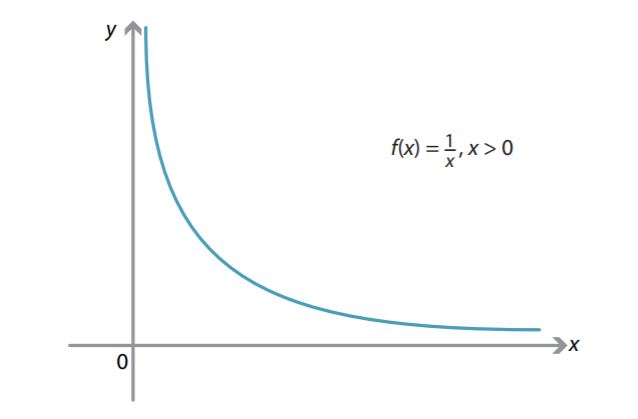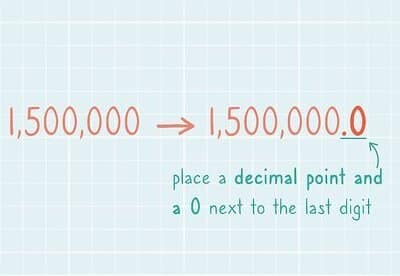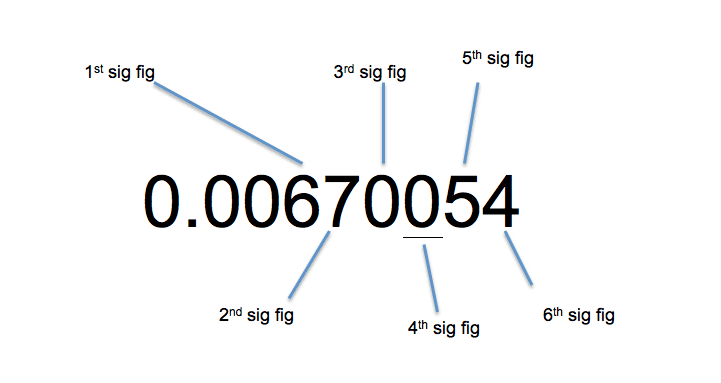# Education BlogHow to write a number, equation, or polynomial in standard form

The standard form of a polynomial is to write down the variable with the highest degree in the first place.Is it Good to Use Calculator Online? – Question & Answer

Online calculators have evolved from simple arithmetic calculators to sophisticated scientific and graphing calculators.An insight into limits: Understanding basic laws of Limits

Limits are used extensively in calculus and that’s why holds utmost importance in mathematics. A limit describes the action of a function near a threshold and operates the behavior of functions.How to Write Numbers in Standard Form

The standard form is also called scientific notation in the United States, a method used to express very large and small numbers in decimal form.